## RS Aggarwal Class 7 Solutions Chapter 9 Unitary Method Ex 9A

These Solutions are part of RS Aggarwal Solutions Class 7. Here we have given RS Aggarwal Solutions Class 7 Chapter 9 Unitary Method Ex 9A.

Other Exercises

Question 1.
Solution:
Cost of 15 oranges = Rs. 110
Cost of 1 orange = Rs. $$\frac { 110 }{ 15 }$$
and cost of 39 oranges = Rs. $$\frac { 110 }{ 15 }$$ x 39
= Rs. 22 x 13 = Rs. 286

Question 2.
Solution:
In Rs. 260, the sugar is bought = 8 kg
and in Re. 1, the sugar is bought = $$\frac { 8 }{ 260 }$$ kg
Then in Rs. 877.50, the sugar will be bought = $$\frac { 8 }{ 260 }$$ x 877.50 kg
= $$\frac { 8 }{ 260 }$$ x $$\frac { 87750 }{ 100 }$$
= 27 kg

Question 3.
Solution:
In Rs. 6290, silk is purchased = 37 m
and in Re. 1, silk is purchased = $$\frac { 37 }{ 6290 }$$ m
and in Rs. 4420, silk will be purchased 37
= $$\frac { 37 }{ 6290 }$$ x 4420 m = 26 m

Question 4.
Solution:
Rs. 1110 is wages for = 6 days.
Re. 1 will be wages for = $$\frac { 6 }{ 1110 }$$ days
and Rs. 4625 will be wages forQuestion 5.
Solution:
In 42 litres of petrol, a car covers = 357 km
and in 1 litre, car will cover = $$\frac { 357 }{ 42 }$$ km
and in 12 litres, car will cover = $$\frac { 357 }{ 42 }$$ x 12 = 102 km

Question 6.
Solution:
Cost of travelling 900 km is = Rs. 2520
and cost of 1 km will be = Rs. $$\frac { 2520 }{ 900 }$$
andcostof360kmwillbe = Rs. $$\frac { 2520 }{ 900 }$$ x 360 = Rs. 1008

Question 7.
Solution:
To cover a distance of 51 km, time is taken = 45 minutes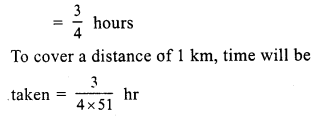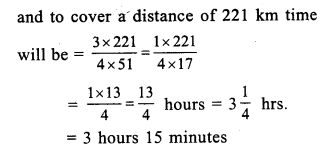Question 8.
Solution:
If weight is 85.5 kg, then length of iron rod = 22.5 m
If weight is 1 kg, then length of rod will be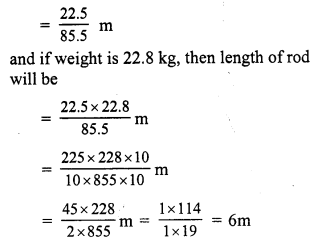Question 9.
Solution:
In 162 grams, sheets are = 6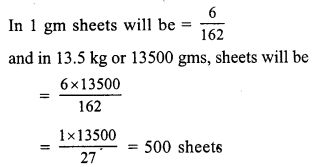Question 10.
Solution:
1152 bars of soap can be packed in 8 cartons
1 bar of soap coil be packed in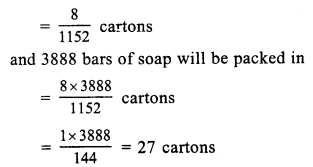Question 11.
Solution:
In 44 mm of thickness, cardboards are = 16
In 1 mm of thickness, cardboards will beQuestion 12.
Solution:
If length of shadow is 8.2 m, then
height of flag staff is = 7 m
If length of shadow is 1 m, then height will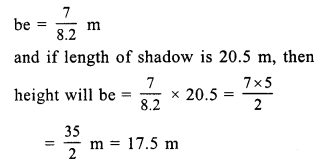Question 13.
Solution:
16.25 m long wall is build by = 15 men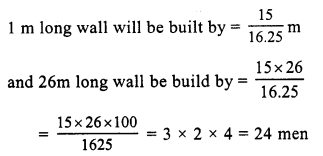Question 14.
Solution:
1350 litres of milk cm be consumed by = 60 patients
1 litres of milk can be consumed by = $$\frac { 60 }{ 1350 }$$ patients
and 1710 litres of milk can be consumed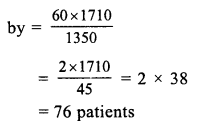Question 15.
Solution:
2.8 cm extension is produced by = 150 g.
1 cm extension will be produced by = $$\frac { 150 }{ 2.8 }$$ g
and 19.6 cm extension will be produced byHope given RS Aggarwal Solutions Class 7 Chapter 9 Unitary Method Ex 9A are helpful to complete your math homework.

If you have any doubts, please comment below. Learn Insta try to provide online math tutoring for you.